Foxtable(狐表)用户栏目专家坐堂 → 麻烦老师帮我看看，哪儿有问题

共有87人关注过本帖树形打印复制链接

# 主题：麻烦老师帮我看看，哪儿有问题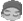yetle
1楼 | 信息 | 搜索 | 邮箱 | 主页 | UC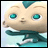加好友发短信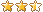sqlload分页已经调试好了，查找不行，查找按钮代码如下：Dim spmc As WinForm.TextBox = e.Form.Controls("商品名称")Dim hh As WinForm.TextBox = e.Form.Controls("货号")'Dim filter As String = "  _identify>0 "Dim filter As String If  spmc.Text > "" Then    filter= filter & " and 商品名称 =  '" & spmc.Text & "'"End IfIf  hh.Text > "" Then    filter= filter & " And 货号 =  '" & hh.Text & "'"End Ifvars("Page") = 1Functions.Execute("LoadData1", filter)Loaddata1代码如下Dim r1 As Integer = (vars("Page") - 1) * 6 + 1 Dim r2 As Integer = vars("Page") * 6 Dim sql As String  sql = "Select * Fr om (Select Row_Number() Over(Order by [业绩] desc) As RowNum, * fr om (select  a.spdm as 货号,b.spmc as 商品名称,avg(a.dj) as 均价, SUM(a.sl) as 业绩,(select  SUM(e.sl)   fr om [14.116.193.208,1588].ERP.dbo.lsthdmx e left join [14.116.193.208,1588].ERP.dbo.lsthd f on  e.djbh=f.djbh where A.spdm=e.spdm and month(f.rq)=datename(month,getdate()) And year(f.rq)=datename(year,getdate()))  as 退货, '\\10.0.0.11\DRP2images\'+b.pic as 图片地址 fr om [14.116.193.208,1588].ERP.dbo.LSXHDMX a left join [14.116.193.208,1588].ERP.dbo.shangpin b on a.spdm=b.spdm left join [14.116.193.208,1588].ERP.dbo.lsxhd c on a.djbh=c.djbh left join [14.116.193.208,1588].ERP.dbo.kehu d on c.dm1=d.khdm where month(c.rq)=datename(month,getdate()) And year(c.rq)=datename(year,getdate())  And d.xzdm=2 And d.lbdm=005 group by a.spdm,b.spmc,b.pic ) as b) As a " sql = sql & " Where RowNum >= " & r1 & " And RowNum <= " & r2 DataTables("商品销售").SQLLoad(sql)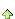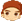2楼 | 信息 | 搜索 | 邮箱 | 主页 | UC加好友发短信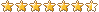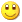Post By：2021/1/14 13:41:00 [只看该作者]

 sql = sql & " Where RowNum >= " & r1 & " And RowNum <= " & r2if args(0) > "" thensql = sql & " and " & args(0)end ifyetle
3楼 | 信息 | 搜索 | 邮箱 | 主页 | UC加好友发短信Post By：2021/1/14 13:56:00 [只看该作者]

 if args(0) > "" thensql = sql & " and " & args(0)end if4楼 | 信息 | 搜索 | 邮箱 | 主页 | UC加好友发短信Post By：2021/1/14 13:58:00 [只看该作者]

 向函数里传递了一个条件做参数，但是从来没有用到：http://www.foxtable.com/webhelp/topics/1486.htmyetle
5楼 | 信息 | 搜索 | 邮箱 | 主页 | UC加好友发短信Post By：2021/1/14 14:39:00 [只看该作者]

 好像有反应了，貌似Loaddata1的sql代码包含group by ,查找的时候提示and附近有错误，这样怎么处理？If  spmc.Text > "" Then    filter= filter & " and 商品名称 =  '" & spmc.Text & "'"End IfIf  hh.Text > "" Then    filter= filter & " And 货号 =  '" & hh.Text & "'"End Ifvars("Page") = 1Functions.Execute("LoadData1", filter) sql = "Select * Fr om (Select Row_Number() Over(Order by [业绩] desc) As RowNum, * fr om (select  a.spdm as 货号,b.spmc as 商品名称,avg(a.dj) as 均价, SUM(a.sl) as 业绩,(select  SUM(e.sl)   fr om [14.116.193.208,1588].ERP.dbo.lsthdmx e left join [14.116.193.208,1588].ERP.dbo.lsthd f on  e.djbh=f.djbh where A.spdm=e.spdm and month(f.rq)=datename(month,getdate()) And year(f.rq)=datename(year,getdate()))  as 退货, '\\10.0.0.11\DRP2images\'+b.pic as 图片地址 fr om [14.116.193.208,1588].ERP.dbo.LSXHDMX a left join [14.116.193.208,1588].ERP.dbo.shangpin b on a.spdm=b.spdm left join [14.116.193.208,1588].ERP.dbo.lsxhd c on a.djbh=c.djbh left join [14.116.193.208,1588].ERP.dbo.kehu d on c.dm1=d.khdm where month(c.rq)=datename(month,getdate()) And year(c.rq)=datename(year,getdate())  And d.xzdm=2 And d.lbdm=005 group by a.spdm,b.spmc,b.pic ) as b) As a "6楼 | 信息 | 搜索 | 邮箱 | 主页 | UC加好友发短信Post By：2021/1/14 14:48:00 [只看该作者]

 原来的sql放到数据库执行有没有问题？yetle
7楼 | 信息 | 搜索 | 邮箱 | 主页 | UC加好友发短信Post By：2021/1/14 14:53:00 [只看该作者]

 不加查询条件的时候没有问题 [此贴子已经被作者于2021/1/14 14:53:49编辑过]8楼 | 信息 | 搜索 | 邮箱 | 主页 | UC加好友发短信Post By：2021/1/14 14:56:00 [只看该作者]

 到命令窗口输出不加条件，和加条件，2个完整的sql发上来看看yetle
9楼 | 信息 | 搜索 | 邮箱 | 主页 | UC加好友发短信Post By：2021/1/14 15:20:00 [只看该作者]

 Dim filter As String 改成Dim filter As String = "  1=1 "就可以了If  spmc.Text > "" Then    filter= filter & " and 商品名称 =  '" & spmc.Text & "'"End IfIf  hh.Text > "" Then    filter= filter & " And 货号 =  '" & hh.Text & "'"End Ifvars("Page") = 1Functions.Execute("LoadData1", filter)谢谢老师，辛苦了10楼 | 信息 | 搜索 | 邮箱 | 主页 | UC加好友发短信Post By：2021/1/14 15:24:00 [只看该作者]

 看最终拼接的sql，不是看代码